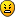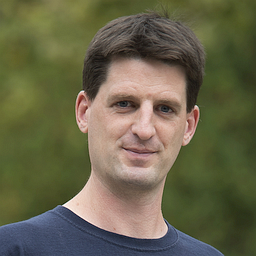cancel
Showing results for
Did you mean:
cancel
Showing results for
Did you mean:
Developer forum

## Face display of a SWEEP commandEnthusiast
Hello,

I am encountering a display issue while using the SWEEP command. Compared to a simple BRICK one, if I am to make a straight extrusion, there is some kind of shading appearing on the faces. However, the base profile is strictly a square and the extrusion is totally vertical with no twist

I tried changing the status codes or the mask but to no effect. Here is the picture of a BRICK (left) and a SWEEP (right)

And both codes
`BRICK LA, LA, HT`
```SWEEP	4, 2, 0, 1, 1+2+4+16+32,
0, 0, 0,
L, 0, 0,
L, L, 0,
0, L, 0,
0, 0, 0,
0, 0, HT```
This does not seem to be an issue while rendering so it's probably just a display issue
1 ACCEPTED SOLUTION
8 REPLIES 8If You add 64*j7 to the mask value then the result is "square"

j1=1! base surface is present,
j2=1! top surface is present,
j3=1! side surface is present,
j5=1! base edges are visible,
j6=1! top edges are visible,
j7=1! cross-section edges are visible, surface is articulated.
mask = j1 + 2*j2 + 4*j3 + 16*j5 + 32*j6 + 64*j7
L=1
HT=3
SWEEP 4, 2, 0, 1, mask,

0, 0, 0,
L, 0, 0,
L, L, 0,
0, L, 0,
0, 0, 0,
0, 0, HT
- AC-24 FIN - WIN 10 - HP Zbook -
“A winner is just a loser who tried one more time.”
George M. Moore, Jr.Enthusiast
Pertti wrote:
If You add 64*j7 to the mask value then the result is "square"

j1=1! base surface is present,
j2=1! top surface is present,
j3=1! side surface is present,
j5=1! base edges are visible,
j6=1! top edges are visible,
j7=1! cross-section edges are visible, surface is articulated.
mask = j1 + 2*j2 + 4*j3 + 16*j5 + 32*j6 + 64*j7
L=1
HT=3
SWEEP 4, 2, 0, 1, mask,

0, 0, 0,
L, 0, 0,
L, L, 0,
0, L, 0,
0, 0, 0,
0, 0, HT
Hello Pertti,

Thanks for the reply. The 64 code appears to fix the smoothing effect however now there are diagonal lines on all side surfaces insteadI tried most combinations for the mask and also changed the twisting values but I do not see any improvement. I'm guessing there is no other choice to have either the smoothing effect or the diagonal lines ?

Just for info, the reason I would like to use the SWEEP instead of a simple BRICK is that I need to parametrically change the top for both Z (delta from ZZYZX) and X (delta from sweep origin) values and that the top would need to still be cut perpendicularly to the side faces.

I tried using EXTRUDE for that but the top was always horizontally cut which was not the desired effect. Maybe I'm not using the proper command and it would be better to use a BRICK and then have some trigonometry to recalculate the base angle dynamicallyOk, there is still one command, "RULED". Why don´t You try it?
- AC-24 FIN - WIN 10 - HP Zbook -
“A winner is just a loser who tried one more time.”
George M. Moore, Jr.Enthusiast
Pertti wrote:
Ok, there is still one command, "RULED". Why don´t You try it?
Because I do not necessarily know the coordinates of the top polygon. If it's only vertical, it's easy but if it's inclined it's not any more. Which is why it was easier to use SWEEP as I could only specify deltas from known values.

But I'll try to do it with BRICK and trigonometry. It seems that's it's the best alternative visually speaking. Thanks for the help.-Still, You could try this formula:
If You define the top surface by 3 points, You get point S height easily.
You can play with the attached object, it is interactive.
```!this formula calculates sz height of a point S(sx,sy) on a plane P1,P2,P3
!3d program

dim Point[][]
Point=1.0
Point=1.0
Point=5.0
Point=1.0
Point=5.0
Point=3.0
Point=1.0
Point=2.1
Point=1.5
sx=2
sy=3

lin_ Point,Point,Point,Point,Point,Point
lin_ Point,Point,Point,Point,Point,Point
lin_ Point,Point,Point,Point,Point,Point

dx1=Point-Point
dy1=Point-Point
dz1=Point-Point

dx2=Point-Point
dy2=Point-Point
dz2=Point-Point

s1=dy1*dz2-dz1*dy2
s2=dz1*dx2-dx1*dz2
s3=dx1*dy2-dy1*dx2

d=-s1*Point-s2*Point-s3*Point
sz=(-s1*sx-s2*sy-d)/s3

- AC-24 FIN - WIN 10 - HP Zbook -
“A winner is just a loser who tried one more time.”
George M. Moore, Jr.Moderator
MetalFingerz wrote:
Just for info, the reason I would like to use the SWEEP instead of a simple BRICK is that I need to parametrically change the top for both Z (delta from ZZYZX) and X (delta from sweep origin) values and that the top would need to still be cut perpendicularly to the side faces.

I tried using EXTRUDE for that but the top was always horizontally cut which was not the desired effect. Maybe I'm not using the proper command and it would be better to use a BRICK and then have some trigonometry to recalculate the base angle dynamicallyI would have a look at TUBE.
It uses the same profile along the path.
You just need to remember that the first and last points on the path are 'dummy' co-ordinates that simple adjust the angle of the start and end faces of the tube.
So you need to allow for these 'dummy' path points to get the correct end face angles.
Basically the last point (that you can't see) is a doubling of the co-ordinates for the second last point (that you can see).
This will set the angle of the last end plane perpendicular to the path.

Barry.

One of the forum moderators.
Versions 6.5 to 25
Dell XPS- i7-6700 @ 3.4Ghz, 16GB ram, GeForce GTX 960 (2GB), Windows 10
Dell Precision 3510 - i7 6820HQ @ 2.70GHz, 16GB RAM, AMD FirePro W5130M, Windows 10
SolutionGraphisoft
With TUBE you will have trouble defining the rotation along the axis. I recommend BRICK and trigonometry to calculate the rotation. You'd have to do the same for a correct TUBE too.
Péter Baksa
Software Engineer, Library as a Platform
Graphisoft SE, BudapestEnthusiast
Peter wrote:
With TUBE you will have trouble defining the rotation along the axis. I recommend BRICK and trigonometry to calculate the rotation. You'd have to do the same for a correct TUBE too.
That's what I ended up doing ultimately. I just had to relearned some trigo which I had forgottenThe result is as intended now and the display of the extrusion looks good. It actually made my code cleaner in the process so overall a good lesson learned from this issue.

Thanks for all the replies, they may help for some other hurdles I may find in the future.Latest solutions

Most liked posts